Reach Us+44-7482-875032
Killing Orbits and Isotropy in General Relativity | OMICS International
Journal of Applied & Computational Mathematics
All submissions of the EM system will be redirected to Online Manuscript Submission System. Authors are requested to submit articles directly to Online Manuscript Submission System of respective journal.

# Killing Orbits and Isotropy in General Relativity

Graham Hall*

Institute of Mathematics, University of Aberdeen, Aberdeen AB24 3UE, Scotland, UK

*Corresponding Author:
Graham Hall
Institute of Mathematics
University of Aberdeen
Aberdeen AB24 3UE
Scotland, UK
E-mail: [email protected]

Received Date: April 23, 2013; Accepted Date: April 24, 2013; Published Date: April 26, 2013

Citation: Hall G (2013) Killing Orbits and Isotropy in General Relativity. J Appl Computat Math 2:e130. doi: 10.4172/2168-9679.1000e130

Copyright: © 2013 Hall G. This is an open-access article distributed under theterms of the Creative Commons Attribution License, which permits unrestricted use, distribution, and reproduction in any medium, provided the original author and source are credited.

Visit for more related articles at Journal of Applied & Computational Mathematics

This brief note is intended to show how a global and rigorous approach to certain problems regarding symmetry in general relativity theory may be given using the theory of Killing orbits and isotropy. Examples from cosmology and plane wave theory are chosen to illustrate the method. More details on these examples may be found in  and on the general techniques used in [2,3].

Let (M, g) be a space-time and let K(M) denote the Lie algebra (under the usual Lie bracket of vector fields) of all global, Killing vector fields on M. Thus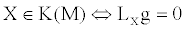X 2 K(M), where L denotes the Lie derivative. In any coordinate domain of M any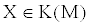satisfies Xa;b=Fab=-Fba where a semi-colon denotes a covariant derivative and F is the Killing bivector associated with X. If TmM denotes the tangent space to M at m let f: K(M) → TmM denote the linear map X→X(m) with range space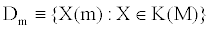and kernel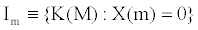Thus dimK(M)=dimDm+dimIm.Im is a subalgebra of K(M) called the isotropy subalgebra and the map D: m→Dm is a generalised distribution on M (that is, it is not necessarily a Frobenius distribution since dimDm may not be constant on M). Since anyis uniquely determined by the values X(m) and F(m) at any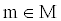it follows that dimK(M) ≤ 10 and that any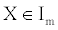is uniquely determined by its associated F(m). It may then be checked that Im is Lie isomorphic to {F(m): F the Killing bivector of someunder the map X → F(m), the latter subalgebra under the Lie product of matrix commutation. It then follows that Im is Lie isomorphic to a subalgebra of the Lorentz algebra L of the Lorentz group and a classification of such subalgebras is well known .

Eachgives rise to a collection of local flow dipheomorphisms dt which are local dipheomorphisms of M and which essentially move each point of some open subset of M a parameter distance t, for t in some open interval of R, along the integral curves of X. Forthe subset Om of points of M to which m may be mapped under successive applications of the permissible dt arising from K(M) is called the orbit (through m) associated with K(M). Such subsets are connected submanifolds of M and are integral manifolds of the distribution D, that is, Dm is tangent to Om at m. The nature of an orbit (null, spacelike or timelike) is the same at all its points. Then dimDm=dimOm and so dimK(M)=dimOm+dimIm for each. Ifand X(m)=0, m is a zero of X and also a fixed point of any dt arising from X, dt (m)=m. Then the linear (pushforward) map ?t* “reflects” the symmetry from X in the tangent space TmM and the other tensor spaces at m.

The dimensions of K(M) and the orbits associated with K(M) can be related in the following way. First an orbit O of K(M) is called dimensionally stable if whenever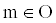there exists a neighbourhood U of m such that the dimension of any orbit through any point of U has the same dimension as O. The following can then be shown. (a) If K(M) admits a 3-dimensional null orbit, 3 ≤ dimK(M) ≤ 7, whilst if K(M) admits any dimensionally stable, 3-dimensional null orbit or any 3-dimensional, non-null orbit (the latter being necessarily dimensionally stable), 3 ≤ dimK(M) ≤ 6. (b) If K(M) admits a 2-dimensional, null (respectively a 2-dimensional non-null) orbit, 2 ≤ dimK(M) ≤ 5, (respectively, 2 ≤ dimK(M) ≤ 4), whilst if K(M) admits any 2-dimensional, dimensionally stable orbit, 2 ≤ dimK(M) ≤ 3. (c) If K(M) admits a 1-dimensional, null (respectively a 1-dimensional non-null) orbit, 1 ≤ dimK(M) ≤ 5, (respectively, 1 dimK(M) ≤ 4), whilst if K(M) admits any 1-dimensional, dimensionally stable orbit, dimK(M)=1. These results, together with the above theory, may be used to give a global approach to some problems with high symmetry and from which the usual results, often obtained by somewhat ad hoc methods, may be derived. Two examples drawn from cosmology and plane wave theory are used to clarify the ideas.

First, consider cosmological models. The concept of isotropy (“all directions are equivalent”) only makes sense if one takes it to mean that all incoming null directions (of photons from observations) are, in some sense, “equivalent”. So call a space-time cosmological if, for eachthe collection of linear maps ?t* arising from members of Im act transitively on the set of null directions at m. This imposes a restriction on the Lie algebra Im and, following a check on the collection of possible subalgebras of L, it turns out that Im can only be Lie isomorphic to o(3) (subalgebra R13 in ) or L itself (subalgebra R15). However, using the theory of the previous paragraphs, it can be shown that one necessarily has the global results that either Im ≈ o(3) at everyor Im ≈ L at everyUsing the results on the relationship between dimK(M) and orbit dimension, one then finds that any orbit of K(M) has dimension 3 or 4 and (M, g) consists of either a single 4-dimensional orbit or each of its orbits is 3-dimensional and spacelike. All orbits are dimensionally stable. Further one easily shows that either (i) Im is isomorphic to L for eachin which case, (M, g) has constant curvature and dimK(M)=10 with K(M) having a single 4-dimensional orbit, or, (ii) Im is isomorphic to so(3) at eachand K(M) has a single 4-dimensional orbit. In this case dimK(M)=7, or, (iii) Im is isomorphic to o(3) for eachand each orbit of K(M) is 3-dimensional and spacelike. In this case dimK(M)=6. If case (i) holds, (M, g) is of constant curvature and hence is locally de Sitter, anti-de Sitter, or Minkowski space whilst if case (ii) holds, (M, g) is either of constant curvature or locally of the Einstein static type. If case (iii) holds, (M, g) admits an open dense subset V such that each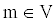admits a neighbourhood whose restricted metric is either of constant curvature or of the (generic) FRWL type. [It is noted that there is no contradiction if (M, g) is of constant curvature and dimK(M)<10; however the local Killing algebra will be 10-dimensional, (see e.g. ) The smoothness of the relevant geometrical objects on M follows from the smoothness of g and the members of K(M). One thus achieves a description of all possible models satisfying the above cosmological condition.

The assumption that (M, g) is cosmological also forces “homogeneity” onto (M, g) in the sense that either there exists a 4-dimensional orbit (transitivity) or the orbits are 3-dimensional and give a local cosmic time function (and in (ii), although the Killing orbit is 4-dimensional, a 6-dimensional subalgebra of K(M) may be shown to exist whose integral manifolds give a local cosmic time function).

One may also apply this method to a study of plane wave symmetry. Let (M, g) be a space-time and let l be a null direction atA wave surface of l at m is a spacelike, 2-dimensional subspace of TmM orthogonal to l. If l, n, x, y is a null tetrad at m, the wave surfaces of l at m can be represented in a one-to-one way (by the pair (a, b) with a,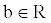) by those subspaces spanned by vectors of the form x+al and y+bl. Each unit timelike vector u at m determines a unique wave surface of l at m which is orthogonal to u (and l) and is then the instantaneous wave surface of l at m for the observer with 4-velocity u at m. Suppose one attempts to propose a definition of a plane wave (M, g) by insisting that the Killing algebra K(M) of (M, g) is such that for eachthere is a unique null direction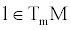such that the ?t* arising from members of Im are transitive on the set of wave surfaces of l at m. One can again check the subalgebras of L for candidates for Im. The situation is a little more complicated now and it turns out that five subalgebras of L are possible and which have dimension 2 or 3. However, in all cases, the maps ?t* fix the direction of l at m and it can be shown that this is the only direction that they fix. Then, as in the cosmological case, one can achieve a global description of all such models. In fact, if one further restricts by the assumptions that all orbits of K(M) are dimensionally stable and that no non-empty open subsets of M of constant curvature exist then one gets the results that either Im is 2-dimensional at each with dimK(M)=5 (respectively, dimK(M)=6) and all orbits are 3-dimensional and null (respectively, there exists a single 4-dimensional orbit), or Im is 3-dimensional at eachwith dimK(M)=6 (respectively, dimK(M)=7) and all orbits are 3-dimensional and null (respectively, there exists a single 4-dimensional orbit). These solutions can then be shown to give the usual (Petrov type N or O) plane wave metrics including, of course, the homogeneous ones and the one with non-zero cosmological constant given in [5,6].

#### References

Select your language of interest to view the total content in your interested language

### Article Usage

• Total views: 12272
• [From(publication date):
August-2013 - Aug 20, 2019]
• Breakdown by view type
• HTML page views : 8482
• PDF downloads : 3790

## Post your commentCan't read the image? click here to refresh
###### Peer Reviewed Journals

Make the best use of Scientific Research and information from our 700 + peer reviewed, Open Access Journals

International Conferences 2019-20

Meet Inspiring Speakers and Experts at our 3000+ Global Annual Meetings

Top